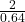## If the gravitational force between a planet of mass M and a satellite of mass m at distance is 2.5 * 10 ^ 3 * N what is the force exerted on

Question

If the gravitational force between a planet of mass M and a satellite of mass m at distance is 2.5 * 10 ^ 3 * N what is the force exerted on a larger satellite of mass 2m , at a distance 0.8r away from planet M ?

in progress 0
2 months 2021-07-31T19:28:49+00:00 1 Answers 1 views 0

F = 7.8  10³ N

Explanation:

For this exercise we will use the law of universal Gravitational

F = G M m / r²

for initial conditions

F₀ = G M m₀ / r₀²

They tell us that the mass changed at m = 2m  distance at r = 0.8r₀

we substitute

F = G M 2m₀ / (0.8 r₀) ²

F = (GM m₀/ r₀²)F = 3.125 F₀

let’s calculate

F = 3.125 2.5 10³

F = 7.8125 10³ N